# Functions

Thinking about teaching functions in the next few weeks (to UK Year 12 ages 16-17) I realised that I could use Desmos to illustrate composite functions; the following slideshow illustrates the syntax.

We can also use Desmos to illustrate a function and its inverse. To create the page below (select the image), I started with a graph already online illustrating the general case of a quadratic function and its inverse and simplified it. f(x) and g(x) can be changed to a different function and its inverse. Note that the domain of f(x) can be changed.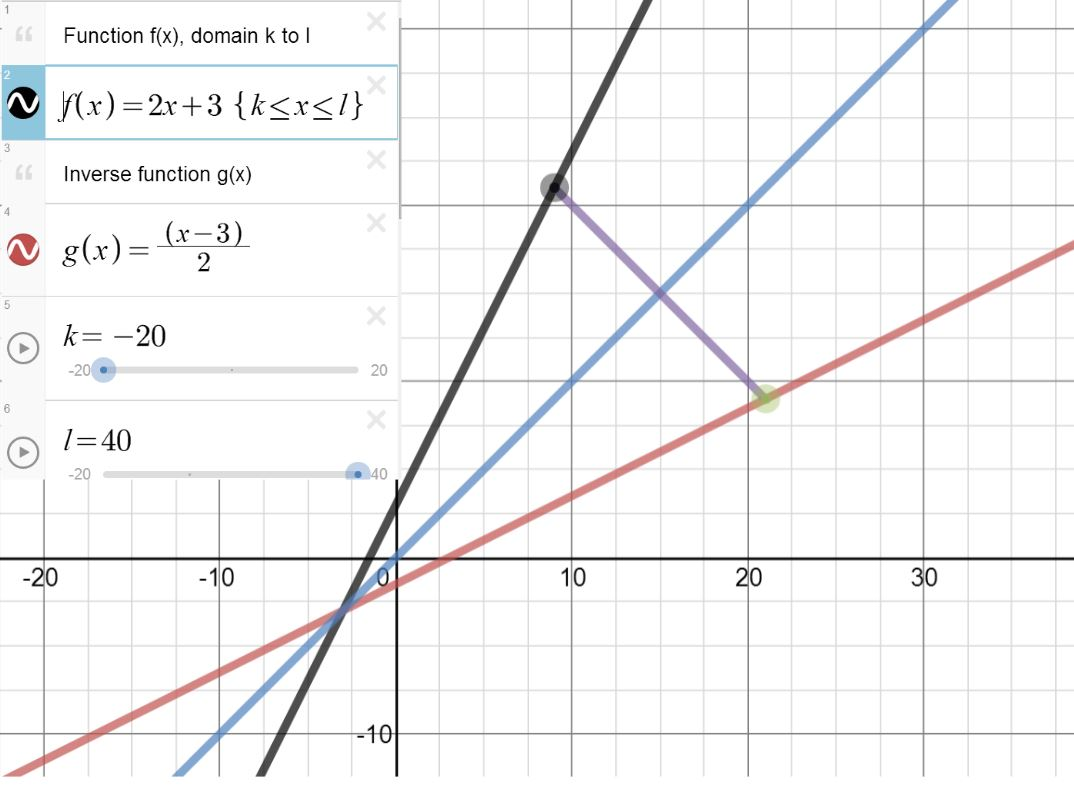Further examples: exponential function and basic quadratic (where we need to restrict the domain for an inverse function to exist).

Staying with Desmos, as I have mentioned before, the function notation is excellent for transformations:

I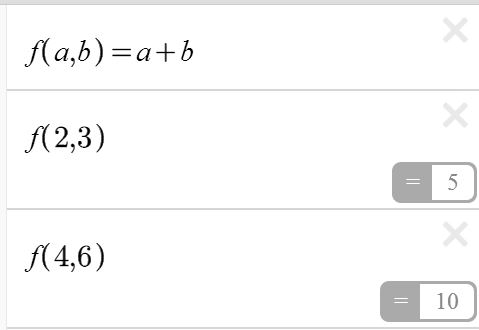t is also possible to define a function with more than one argument and use Desmos as a calculator

For some clear examples and a resource to point students to, Functions from The University of Plymouth Mathematics Support Materials is useful. The format used in this series makes the examples clear and all the exercises given have answers.

Other useful resources (requires Java) include the Wisweb applets, algebra arrows could be used to demonstrate functions and their inverses as shown in the following images.

This slideshow requires JavaScript.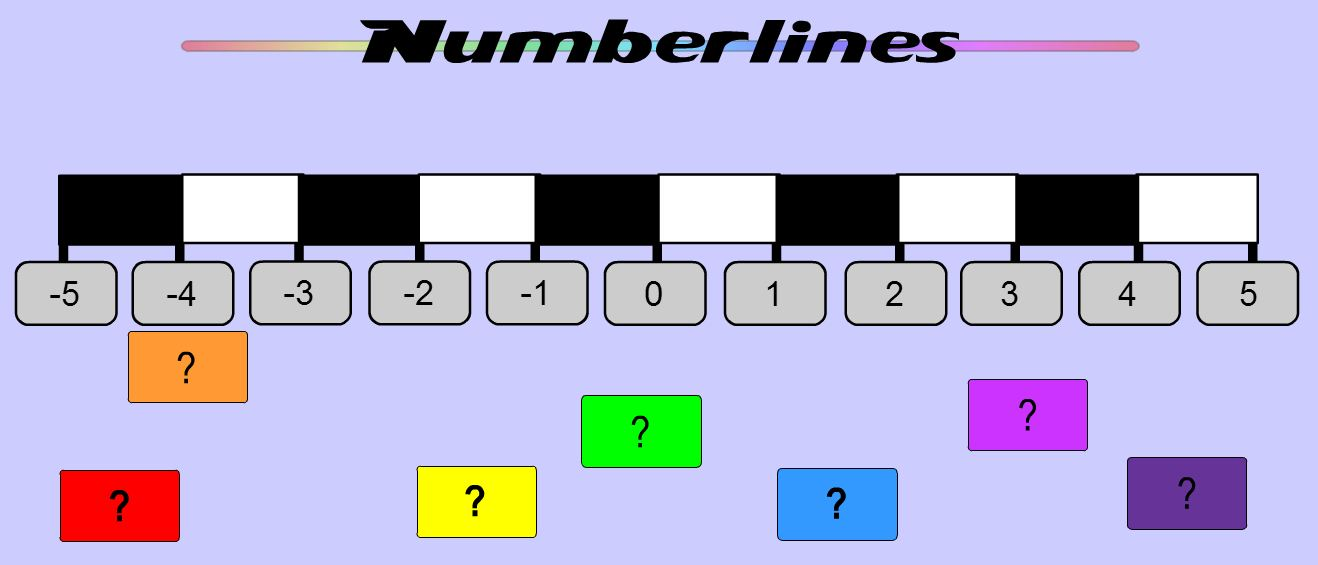# Negative Numbers – Resources

Sometimes resources for younger students can be useful for lower secondary age students, see for example Mark Robinson’s Numberlines  from the old Ambleside Primary School site which includes an option to display a number line from -5 to 5.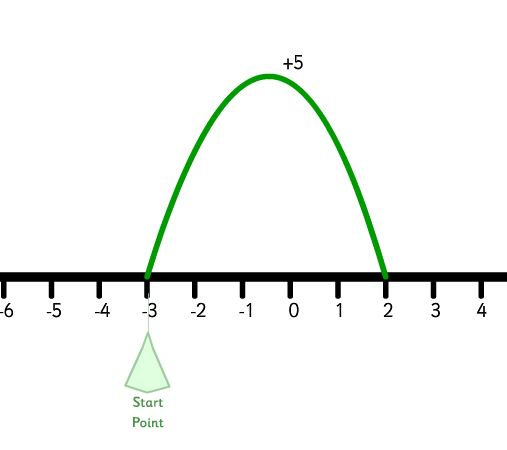For another excellent number line resource see J Barrett’s Numberline Jump Maker on ictgames.com. I often recommend that students sketch a number line to help with addition and subtraction problems and very clear resources like these can really help. Teacher Resources on Line includes a Big Number Line under Basic Materials for display on a classroom wall

Games can be an excellent way to practise with negative numbers see for example games such as Connect 3 from Nrich and Tic Tac Go, a Wisweb applet.

Further resources include exercises from Trinity School in Nottingham (under Number) and Interactive Resources from CIMT (see unit 3, 3.3 on Negative Numbers and Unit 15, 15.1 and 15.2 for operations with negative numbers in the tutorials section).

There are many excellent resources on TES, the resource collections includes a section on Topic Specials which includes 10 of my favourite resources on Negative Numbers.

# Solving Equations

A collection of resources to use to demonstrate and practise solving equations.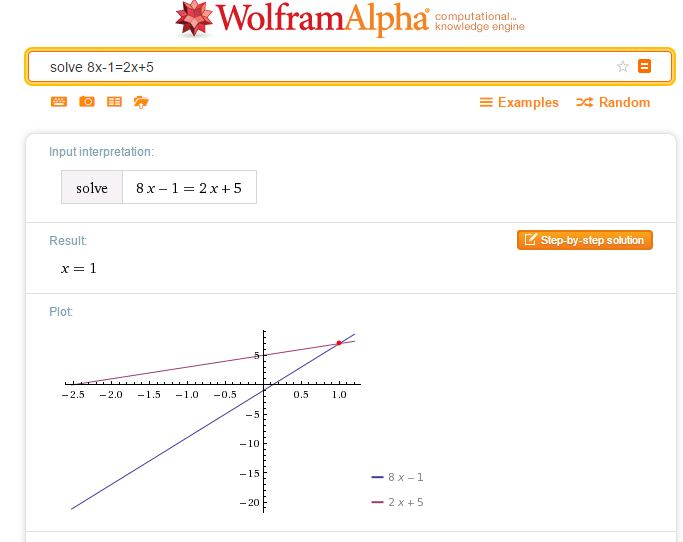A simple way to check a solution to an equation of any type is to simply enter your equation as a WolframAlpha query. Note that WolframAlpha includes a graphical illustration; it is so important for students to understand how equations may be solved graphically; I always illustrate graphical solutions when we are working with Algebra to help students make these links. Desmos of course, is ideal here.The following resources allow practice in choosing the correct operations to reach a solution.

Centre for Innovation in Mathematics Teaching
CIMT
have tutorials on equations: Linear Equations 1, Linear Equations 2, and Linear Equations with Brackets in their Interactive ResourcesChoose the type of equation you require then the sequence of operations required to solve the equation.
Select Do it after each operation, for example -32 Do it were the keys selected to start the above problem.

The next sites work in a similar way to the subtangent resource.

Flashy MathsSolving Equations (select the swf file to play online)There are 4 levels to choose from: the level 1 equations are of the type ax = b,
level 2, x±c = d, level 3, ex±f = g and level 4, hx±i = hx±j = k.
Choose the series of operations you require to solve the equation selecting apply at each stage to see the result of your chosen operation.
Choose numbers to add and subtract integers and letters to add and subtract variables.

MathsNetEquation Buster
Playing at level 5 introduces equations with fractions.From the National Library of Virtual Manipulatives try Algebra Balance Scales and Algebra Balance Scales – Negatives.
Note that these manipulatives allow you to create a problem of your own (so long as it has an integer solution) as well as solving problems given.From WaldoMaths, try this applet on solving simple linear equations and this on equations requiring more complex manipulation.
Each applet has several levels.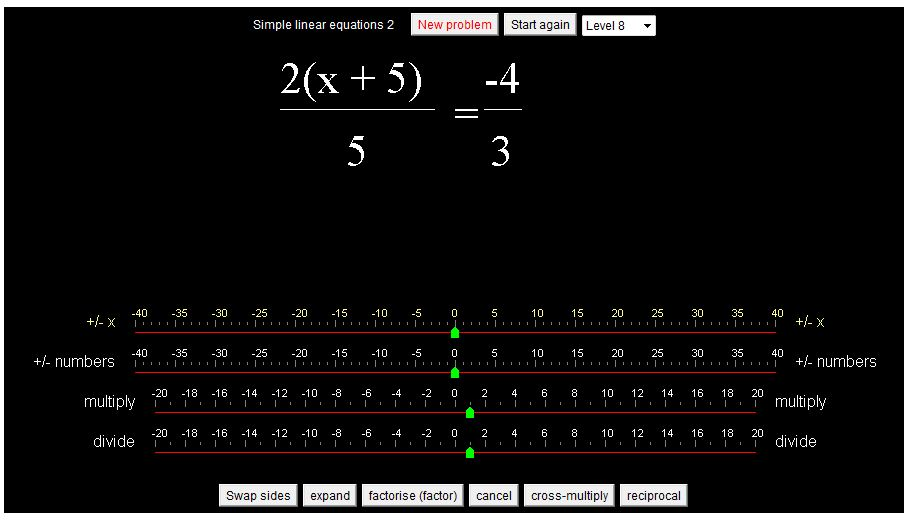Unlike the other resources this allows the user to enter the equation resulting from the chosen operation.
The solution is checked at each stage.
As well as solving the given problems (20 problems) the user can select ‘Make an equation yourself’ to enter any equation then solve that equation.
(Unlike the NLVM resource above the x can be any real number).

The following video shows the keystrokes required to enter and solve an equation.